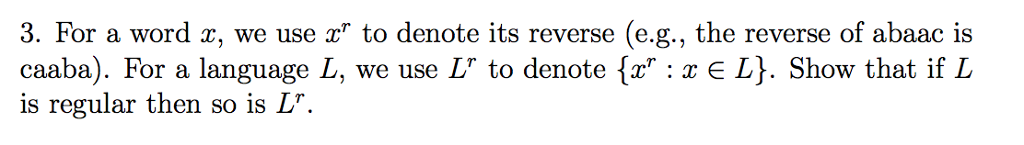# Question & Answer: For a word x, we use x^r to denote its reverse (e.g., the reverse of abaac is caaba). For a…..For a word x, we use x^r to denote its reverse (e.g., the reverse of abaac is caaba). For a language L, we use L^r to denote {x^r: x elementof L}. Show that if L is regular then so is L^r.

Don't use plagiarized sources. Get Your Custom Essay on
Question & Answer: For a word x, we use x^r to denote its reverse (e.g., the reverse of abaac is caaba). For a…..
GET AN ESSAY WRITTEN FOR YOU FROM AS LOW AS \$13/PAGE

Hi,

Given

reverse for a word x= x^r

Reverse of a language L = L^r where {x^r belongs to L}

It is one of the topic in finite automata, from above statements we can say that if L can be written as a regular expression , then L^r can also be written as a regular expression.

Regular expression is built from smaller one by union , concatenation and Kleen star.

Also we can consider the closure properties of regular languages unse simple set operations .

First through Union :

.

Say a word x is a1a2a3…an , reversal of it is written backwards

We use x^R for reversal of word x , each  is in L(F). The reversal of a string in L(F^*) . Which is in L(E).